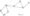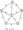# Analysis and Design of Algorithms Aug 2004

Note: Answer any FIVE full questions.

1. (a) Define 0,0), O notations.

(b) Prove : 3n3-f 2n2 —0(n2); 3n ^ 0(2n)

(c) If ^(n) = 0(/(n)) and T2(rc) = 0(#(n)), then show that Ti(n-)-r T2(n) ~ 0(max(f(n),ff(n))).

2.  (a) Write the bubble sort algorithm and show that the worst case efficiency is quadratic.

(b) Outline an exhaustive search algorithm for travelling salesman problem. What is the efficiency class of this algorithm? Illustrate vyith an example.

3. (a) Describe a binary search algorithm. Show that the worst case efficiency is in \$)(log 77,).

(b) Write algorithms for preorder, postorder, and inorder traversals of a tree. List the tree of Fig 3(b) in preorder, postorder and inorder.4. (a) Write DFS algorithm and find its worst case efficiency.

(b) Write an algorithm to topologically sort a diagraph using DFS. Prove the correctness and find the time efficiency.

(c)      Topologically sort the following graph shown in Fig.4(c)5. (a) What is a 2-3 tree? Explain search, insertion and deletion operations and show that these operations are all in (log n) in both worst and average case, do

(b) What is a heap? Outline an algorithm to construct a heap. Derive the efficiency class of this algorithm.

6.  (a) Describe an algorithm with an example to compute binomial coefficient and derive its time efficiency.

(b) Design a, @ (n2) algorithm for finding an optimal binary search tree.

7. (a) Write the Kruskal’s algorithm to find minimum spanning tree in time

0(| E | log | E j); where j E | is the number of edges. Prove the correctness and derive the efficiency class of the algorithm.

(b) Apply Kruskars algorithm to find minimum spanning tree of the graph shown in Fig.7(b).8 (a)   Define Pr NP, and NP-complete problems.

(b) Write twice around the tree approximation algorithm for travelling salesman problem. Illustrate the working of the algorithm with an example.

(c) Show that the above algorithm is a 2 approximation algorithm.